Home > CCA2 > Chapter 8 > Lesson 8.3.1 > Problem8-125

8-125.
1. Consider the equation 5x2 − 7x − 6 = 0 as you answer the questions in parts (a) through (d) below. Homework Help ✎

1. What are the factors of 5x2 − 7x − 6?

2. What are the solutions to the equation?

3. Explain the relationship between the factors of the polynomial expression and the solutions to the equation.

4. How are the solutions to the equation related to the lead coefficient and constant term in the original polynomial?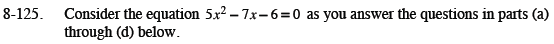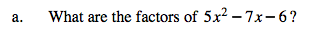Watch the video below if you need help remembering how to factor quadratics. If you only need a little help, jump to 2:28 in the video. Click the following link for a full version: Factoring Quadratics Video

(5x + 3)(x − 2)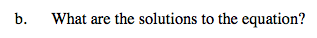Use the Zero Product Property.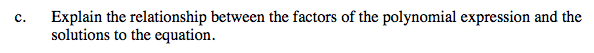The solutions are the values that make the factors equal to 0.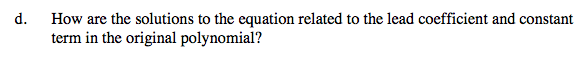3 and 2 are factors of 6 while 5 is a factor of the lead coefficient.